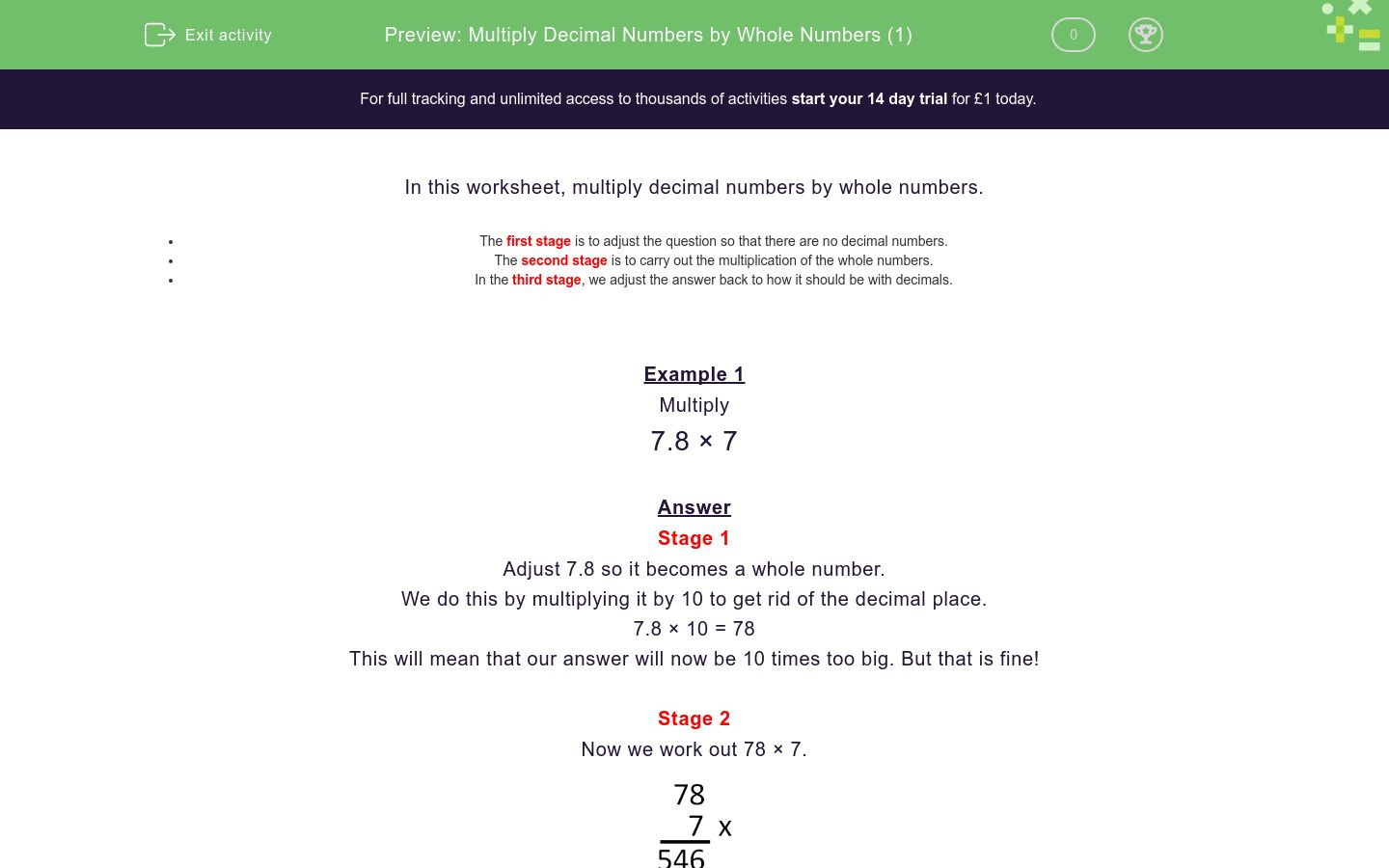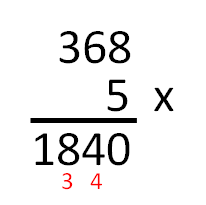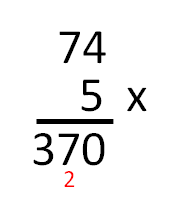# Multiply Decimal Numbers by Whole Numbers (1)

In this worksheet, students multiply one-digit numbers with up to two decimal places by whole numbersKey stage:  KS 2

Curriculum topic:  Number: Fractions, Decimals and Percentages

Curriculum subtopic:  Multiply with Two Decimal Places

Difficulty level:### QUESTION 1 of 10

In this worksheet, you must multiply decimal numbers by whole numbers.

• The first stage is to adjust the question so that there are no decimal numbers.
• The second stage is to carry out the multiplication of the whole numbers.
• In the third stage, we adjust the answer back to how it should be with decimals.

Example 1

Multiply

7.8 × 7

Stage 1

Adjust 7.8 so it becomes a whole number.

We do this by multiplying it by 10 to get rid of the decimal place.

7.8 × 10 = 78

This will mean that our answer will now be 10 times too big. But that is fine!

Stage 2

Now we work out 78 × 7.Stage 3

Now, remember that this is 10 times too big.

So we divide 546 ÷ 10 = 54.6.

7.8 × 7 = 54.6

Example 2

Multiply

3.68 × 5

Stage 1

Adjust 3.68 so it becomes a whole number.

We do this by multiplying it by 100 to get rid of the 2 decimal places.

3.68 × 100 = 368

This will mean that our answer will now be 100 times too big. But that is fine!

Stage 2

Now we work out 368 × 5.Stage 3

Now, remember that this is 100 times too big.

So we divide 1840 ÷ 100 = 18.40 = 18.4.

3.68 × 5 = 18.4

Multiply

3.2 × 4

Multiply

2.3 × 4

Multiply

3.5 × 3

Multiply

6.2 × 5

Multiply

7.4 × 5

Multiply

2.32 × 3

Multiply

2.32 × 4

Multiply

2.23 × 4

Multiply

2.53 × 5

Multiply

4.32 × 6

• Question 1

Multiply

3.2 × 4

12.8
EDDIE SAYS
Multiply 3.2 by 10 to getDivide answer by 10.
• Question 2

Multiply

2.3 × 4

9.2
EDDIE SAYS
Multiply 2.3 by 10 to getDivide answer by 10.
• Question 3

Multiply

3.5 × 3

10.5
EDDIE SAYS
Multiply 3.5 by 10 to getDivide answer by 10.
• Question 4

Multiply

6.2 × 5

31
31.0
EDDIE SAYS
Multiply 6.2 by 10 to getDivide answer by 10.
• Question 5

Multiply

7.4 × 5

37
37.0
EDDIE SAYS
Multiply 7.4 by 10 to getDivide answer by 10.
• Question 6

Multiply

2.32 × 3

6.96
EDDIE SAYS
Multiply 2.32 by 100 to getDivide answer by 100.
• Question 7

Multiply

2.32 × 4

9.28
EDDIE SAYS
Multiply 2.32 by 100 to getDivide answer by 100.
• Question 8

Multiply

2.23 × 4

8.92
EDDIE SAYS
Multiply 2.23 by 100 to getDivide answer by 100.
• Question 9

Multiply

2.53 × 5

12.65
EDDIE SAYS
Multiply 2.53 by 100 to getDivide answer by 100.
• Question 10

Multiply

4.32 × 6

25.92
EDDIE SAYS
Multiply 4.32 by 100 to getDivide answer by 100.
---- OR ----

Sign up for a £1 trial so you can track and measure your child's progress on this activity.

### What is EdPlace?

We're your National Curriculum aligned online education content provider helping each child succeed in English, maths and science from year 1 to GCSE. With an EdPlace account you’ll be able to track and measure progress, helping each child achieve their best. We build confidence and attainment by personalising each child’s learning at a level that suits them.

Start your £1 trial